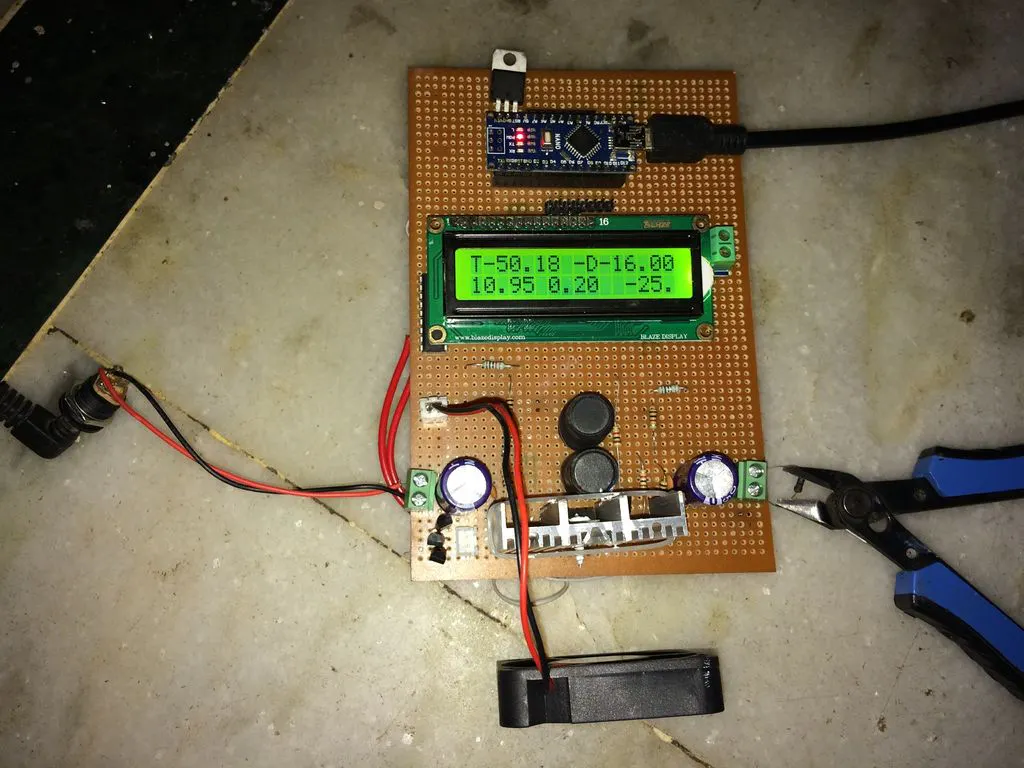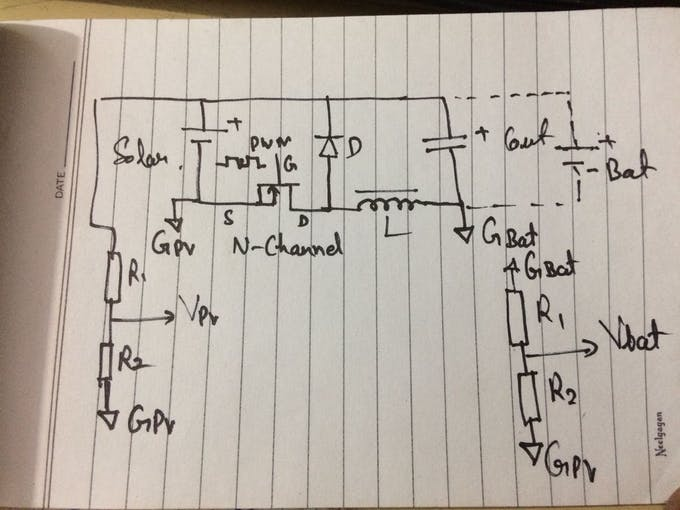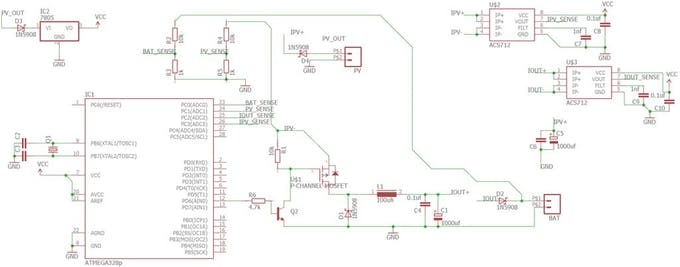Project tutorialArduino - PV MPPT Solar Charger © GPL3+

There are many charge controllers available, but ordinary cheap ones are not efficient enough to use with maximum power from solar panels.

• 51,859 views
• 59 respects

Necessary tools and machinesSoldering iron (generic)

Apps and online services

There are many charge controllers available in market, but ordinary cheap charge controllers are not efficient for use with maximum power from solar panels. And the ones which are efficient are very costly.

So I decided to make my own charge controller to be efficient and smart enough to understand the battery needs and solar conditions. It takes appropriate actions to draw maximum available power from solar and put it inside the battery very efficiently.

If you like my efforts, then please vote for this tutorial.

Step 1: What Is MPPT and Why Do We Need It?

Our solar panels are dumb and not smart enough to understand the battery conditions. Assume we have a 12v/100 watt solar panel and it'll give an output between 18V-21V depending upon manufactures, but batteries are rated for 12v nominal voltage. At full charge conditions they will be 13.6v and will be 11.0v at full discharge. Now let's assume our batteries are at 13v charging, panels are giving 18v, 5.5A at 100% working efficiency (not possible to have 100% but let's assume). Ordinary controllers have a PWM voltage regulator ckt which drops the voltage to 13.6 without any gain in current. It only provides protection against over charging and leakage current to panels during nights.

So we have 13.6v*5.5A = 74.8 watt. We loose approx 25 watt.

To counter this issue, I have used smps buck converter. This kind of converter has above 90% efficiency. Even 90% is considered poor.

Second problem that we have is non-linear output of solar panels. They need to be operated at a certain voltage to harvest maximum available power. Their output varies through the day.

To solve this issue MPPT algorithms are used. MPPT (Maximum Power Point Tracking) as the name suggests this algorithm tracks the maximum available power from panels and varies the output parameters to sustain the condition.

So by using MPPT our panels will be generating maximum available power and buck converter will be putting this charge efficiently into batteries.

Step 2: How Does MPPT Work?

I'm not going to discuss this in detail, so if you want to understand it, have a look at this link - What is MPPT?

In this project I have tracked the input V-I characteristics and output V-I, also. By multiplying the input V-I and output V-I we can have the power in watts.

Let's say we have 17 V 5 A, i.e. 17x5 = 85 watt, at any time of the day. At the same time our output is 13 V 6A, i.e. 13x6 = 78 Watt.

Now MPPT will increase or decrease the output voltage to by comparing to previous input/output power.

If the previous input power was high and the output voltage was lower than present, then output voltage will be lowered down again to get back to high power. And if the output voltage was high, then present voltage will be increased to the previous level. Thus it keeps oscillating around the maximum power point. These oscillations are minimized by efficient MPPT algorithms.

Step 3: Implementing MPPT on Arduino

This is the brain of this charger. Below is the Arduino code to regulate the output and implementing MPPT in a single code block.

// Iout = output current
// Vout = output voltage
// Vin = input voltage
// Pin = input power, Pin_previous = last input power
// Vout_last = last output voltage, Vout_sense = present output voltage
void regulate(float Iout, float Vin, float Vout) {<br>  if((Vout>Vout_max) || (Iout>Iout_max) || ((Pin>Pin_previous && Vout_sense<Vout_last) || (Pin<Pin_previous && Vout_sense>Vout_last)))
{
if(duty_cycle>0)
{
duty_cycle -=1;
}
analogWrite(buck_pin,duty_cycle);
}
else if ((Vout<Vout_max) && (Iout<Iout_max) && ( (Pin>Pin_previous && Vout_sense>Vout_last) || (Pin<Pin_previous && Vout_sense<Vout_last)))
{
if(duty_cycle<240)
{<br>    duty_cycle+=1;
}
analogWrite(buck_pin,duty_cycle);
}
Pin_previous = Pin;
Vin_last = Vin;
Vout_last = Vout;
}

Step 4: Buck Converter

I have used N-channel mosfet to make the buck converter. Usually people choose P-channel mosfet for high side switching and if they choose N-channel mosfet for the same purpose than a driver IC will be required or boot strapping ckt.

But I modified the buck converter ckt to have a low side switching using N-channel mosfet. I'm using N-channel because these are low cost, high power ratings and lower power dissipation. This project uses IRFz44n logic level mosfet, so it can be directly drive by an Arduino PWM pin.

For higher load current, one should use a transistor to apply 10V at gate to get the mosfet into saturation completely and minimize the power dissipation. I have done the same.

As you can see in ckt above, I have placed the mosfet on -ve voltage, thus using +12v from panel as ground. This configuration allows me to use a N-channel mosfet for buck converter with minimum components.

But it also has some drawbacks. As you have both sides -Ve voltage separated, you don't have a common reference ground anymore. So measuring of voltages are very tricky.

I have connected the Arduino at solar input terminals, using its -Ve line as ground for Arduino. We can easily measure the input voltage at this point by using a voltage divider ckt as per our requirement. But we can't measure the output voltage so easily as we don't have a common ground.

Now to do this there is a trick. Instead of measuring the voltage across the output capacitor, I have measured the voltage between two -Ve lines. Using solar -Ve as ground for the Arduino and output -Ve as the signal/voltage to be measured. The value that you got with this measurement should be subtracted from the input voltage measured and you will get the real output voltage across output capacitor.

Vout_sense_temp=Vout_sense_temp*0.92+float(raw_vout)*volt_factor*0.08; //measure volatge across input gnd and output gnd.<br>
Vout_sense=Vin_sense-Vout_sense_temp-diode_volt; //change voltage difference between two grounds to output voltage..

For current measurements I have used ACS-712 current sensing modules. They have been powered by Arduino and connected to input GND.

Internal timers are modified to gain 62.5 Khz PWM at pin D6, which is used to drive the MOSFET. An output blocking diode will be required to provide reverse leakage and reverse polarity protection use Schottky diode of desired current rating for this purpose. The value of inductor depends upon frequency and output current requirements. You can use online available buck converter calculators or use 100uH 5A-10A load. Never exceed the maximum output current of inductor by 80%-90%.

Step 5: Final Touch Up

You can also add additional features to your charger. Mine have LCD to display the parameters and 2 switches to take input from the user.

I'll update the final code and complete ckt diagram very soon.

Step 6: Actual Circuit Diagram, BOM & Code

UPDATE:

I have uploaded the code, bom and circuit. It's slightly different from mine, because it's easier to make this one.

Code

Solar_charger_tutorial_code.inoArduino
///////////////////////////////////////////////////////////////////////////////////////
///////////////////////////////////////////////////////////////////////////////////////
//THE SOFTWARE IS PROVIDED "AS IS", WITHOUT WARRANTY OF ANY KIND, EXPRESS OR
//IMPLIED, INCLUDING BUT NOT LIMITED TO THE WARRANTIES OF MERCHANTABILITY,
//FITNESS FOR A PARTICULAR PURPOSE AND NONINFRINGEMENT. IN NO EVENT SHALL THE
//AUTHORS OR COPYRIGHT HOLDERS BE LIABLE FOR ANY CLAIM, DAMAGES OR OTHER
//LIABILITY, WHETHER IN AN ACTION OF CONTRACT, TORT OR OTHERWISE, ARISING FROM,
//OUT OF OR IN CONNECTION WITH THE SOFTWARE OR THE USE OR OTHER DEALINGS IN
//THE SOFTWARE.
///////////////////////////////////////////////////////////////////////////////////////

#include <LiquidCrystal.h>
#include <avr/wdt.h>
#include <EEPROM.h>
#define vin_pin A1
#define vout_pin A0
#define iout_pin A2
#define iin_pin A3
#define lm35 A4
#define fan 5
#define buck_pin 6
#define button 2
#define led 13
#define charge_led A5
#define light 4

uint8_t auto_mode= 1;
float Pin=0,Pout=0,Pin_previous=0;
float efficiency=0.0;
int raw_vin=0, raw_vout=0, raw_iout=0,raw_iin=0, raw_lm35=0;
float Vout_boost=14.5,Vout_max=15.0, Iout_max=5.0, Vout_float=13.5, Iout_min=0.00,Vin_thresold=10.0;
float Iout_sense,Iin_sense,Iin;
float Vout_sense,Vin_last;
float heat_sink_temp;
float Vin_sense;
uint8_t duty_cycle = 0;
float volt_factor = 0.05376; //change this value to calibrate voltage readings...
String mode="";
bool startup=true, lcd_stat=true,charge=true,mppt_init = true;
unsigned int count=0;

LiquidCrystal lcd(7, 8, 9, 10, 11, 12);
void lcd_show(String data,int column, int row);
void UI();
void set_limits(int cmd,int temp);
void mem_write();
void mppt();
void setup() {
wdt_disable();
watchdogSetup();
// put your setup code here, to run once:
Serial.begin(115200);
pinMode(light,OUTPUT);
pinMode(charge_led,OUTPUT);
digitalWrite(charge_led,LOW);
digitalWrite(light,HIGH);
pinMode(led,OUTPUT);
pinMode(fan,OUTPUT);
pinMode(button,INPUT);
digitalWrite(button,HIGH);
TCCR0B = TCCR0B & 0b11111000 | 0x01; / set pwm at Max... 62.5 Khz
analogWrite(buck_pin,0);
lcd.begin(16,2);
lcd_show("Solar Charger",0,0);
delay(64000);
wdt_reset();
delay(64000);
wdt_reset();
lcd_show("Vi    Vb    Ib  ",0,0);
//////////////////

for(int i=0;i<10;i++) {
delay(2);
}
raw_iout=raw_iout/10;
raw_iin=raw_iin/10;
raw_vout=raw_vout/10;
raw_vin=raw_vin/10;
Iout_sense=float(raw_iout)*5/1023/0.066;
Iin_sense=float(raw_iin)*5/1023/0.066;
Vout_sense_temp=float(raw_vout)*volt_factor;
Vin_sense=float(raw_vin)*volt_factor;
// heat_sink_temp = raw_lm35*0.48; // 0.0049*1000/10
// heat_sink_temp = heat_sink_temp-273.15; //uncomment if using LM235

}

//////////
void watchdogSetup(void)
{
cli();  // disable all interrupts
wdt_reset(); // reset the WDT timer
// Enter Watchdog Configuration mode:
WDTCSR |= (1<<WDCE) | (1<<WDE);
// Set Watchdog settings:
WDTCSR = (1<<WDIE) | (1<<WDE) | (1<<WDP3) | (0<<WDP2) | (0<<WDP1) | (1<<WDP0);
sei();
}
///////
ISR(WDT_vect) // Watchdog timer interrupt.
{
Serial.println(("WDT reset."));
asm volatile (" jmp 0");
// Include your code here - be careful not to use functions they may cause the interrupt to hang and
// prevent a reset.
}
/////

////This function provides various regulations and MPPT implementation...
void regulate(float Iout, float Vin, float Vout) {
mode="";
mode="Buck mode";
if((Vout>Vout_max) || (Iout>Iout_max) || ((Pin>Pin_previous && Vin_sense<Vin_last) || (Pin<Pin_previous && Vin_sense>Vin_last))) {
if(duty_cycle>0) {
duty_cycle-=1;
}
analogWrite(buck_pin,duty_cycle);
}
else if((Vout<Vout_max) && (Iout<Iout_max) && ((Pin>Pin_previous && Vin_sense>Vin_last) || (Pin<Pin_previous && Vin_sense<Vin_last))) {
if(duty_cycle<250) {
duty_cycle+=1;
}
analogWrite(buck_pin,duty_cycle);
}
Pin_previous = Pin;
Vin_last = Vin;
}

void auto_cutoff(float Iout,float Vin, float Vout){
////////////
if(Vout<=Vout_float && Iout>Iout_min+1){
charge = true;
}
/////////////
if((Vout>Vout_max) && (Iout<Iout_min) && (charge==true)) {
charge = false;
Serial.println("Charging Completed.");
digitalWrite(led,HIGH);
digitalWrite(charge_led,LOW);
}
else if(Vin<Vin_thresold) {
duty_cycle=0;
analogWrite(buck_pin,duty_cycle);
Serial.println("LOW Input Voltage.");
lcd_show("Input Volt. Low  ",0,1);
wdt_reset();
for(int i=0;i<10;i++){
digitalWrite(led,HIGH);
digitalWrite(charge_led,LOW);
delay(6000);
digitalWrite(charge_led,HIGH);
digitalWrite(led,LOW);
delay(6000);
}
wdt_reset();
}
else if(heat_sink_temp>80.0){
duty_cycle=0;
analogWrite(buck_pin,duty_cycle);
Serial.println("Over Heat Shutdown");
lcd_show("Over Heat Fail  ",0,1);
wdt_reset();
for(int i=0;i<10;i++){
digitalWrite(led,HIGH);
digitalWrite(charge_led,LOW);
delay(4000);
digitalWrite(charge_led,HIGH);
digitalWrite(led,LOW);
delay(4000);
}
wdt_reset();
}
else {
charge = true;
digitalWrite(charge_led,HIGH);
regulate(Iout_sense, Vin_sense, Vout_sense);
digitalWrite(led,LOW);
}
}

void soft_start() {
for(int i=0;i<20;i++) {
regulate(Iout_sense, Vin_sense, Vout_sense);
Serial.print("Vin= ");Serial.println(Vin_sense);
Serial.print("Vout= ");Serial.println(Vout_sense);
Serial.print("Iout= ");Serial.println(Iout_sense);
Serial.print("Duty cycle= ");Serial.println(duty_cycle);
Serial.print("Charger MODE : ");Serial.println(mode);
Serial.println("Soft Start Activated");
delay(32000);
}

startup=false;
mppt_init = false;
}

void lcd_show(String data,int column, int row) {
lcd.setCursor(column,row);
if(data.length()>0) {
for(int i=0;i<data.length();i++) {
lcd.print(" ");
}
lcd.setCursor(column,row);
lcd.print(data);
}
}

void lcd_num(float num,int column, int row){
lcd.setCursor(column,row);
for(int i=0;i<6;i++) {
lcd.print(" ");
}
lcd.setCursor(column,row);
lcd.print(num);
}

void UI(){
int cmd=0,i=0 ;
while(i==0){
wdt_reset();
cmd+=1;
delay(64000);
}
switch(cmd){
case 1:
lcd_show("Vb Boost=",0,0);
lcd_num(Vout_boost,9,0);
wdt_reset();
Vout_boost+=0.02;
if(Vout_boost>17.0){
Vout_boost=0;
}
delay(8000);
lcd_num(Vout_boost,9,0);
}}
break;
case 2:
lcd_show("Vb Float=",0,0);
lcd_num(Vout_float,9,0);
wdt_reset();
Vout_float+=0.02;
if(Vout_float>17.0){
Vout_float=0;
}
delay(8000);
lcd_num(Vout_float,9,0);
}
}
break;
case 3:
lcd_show("Ib max.= ",0,0);
lcd_num(Iout_max,9,0);
wdt_reset();
Iout_max+=0.05;
if(Iout_max>20.0){
Iout_max=0;
}
delay(8000);
lcd_num(Iout_max,9,0);
}
}
break;
case 4:
lcd_show("Ib min.= ",0,0);
lcd_num(Iout_min,9,0);
wdt_reset();
Iout_min+=0.05;
if(Iout_min>20.0){
Iout_min=0.0;
}
delay(8000);
lcd_num(Iout_min,9,0);
}
}
break;
case 5:
lcd_show("Vin min.= ",0,0);
lcd_num(Vin_thresold,9,0);
wdt_reset();
Vin_thresold+=0.02;
if(Vin_thresold>18.0){
Vin_thresold=0;
}
delay(8000);
lcd_num(Vin_thresold,9,0);
}
}
break;

case 6:
lcd_show("Choose Mode     ",0,0);
lcd_show("Auto Mode       ",0,1);

wdt_reset();
auto_mode=~auto_mode;
if(auto_mode){
lcd_show("Auto Mode ON    ",0,1);
}
else {
lcd_show("Auto Mode OFF   ",0,1);
}
delay(32000);
}
}
break;

case 7:
lcd_show("Press Men+u to   ",0,0);
lcd_show("Save & Exit.     ",0,1);

wdt_reset();
}
//Save Setting to EEPROM.
mem_save();
setup();
i=1;
break;

}
}  }
////////
void mem_save(){
uint8_t value=0;
for(int i =0;i<7;i++){
EEPROM.write(i,0);
}
value = Vout_boost*10;
EEPROM.write(1,value);
value = Vout_float*10;
EEPROM.write(2,value);
value = Iout_max*10;
EEPROM.write(3,value);
value = Iout_min*10;
EEPROM.write(4,value);
// EEPROM.write(5,auto_mode);
value = Vin_thresold*10;
EEPROM.write(6,value);
}
/////

for(int i =0;i<7;i++){
}
}
void set_limits(int cmd,int temp){
switch(cmd) {
case 1:
Vout_boost=float(temp)/10;
Serial.print("Vout_boost= ");
Serial.println(Vout_boost);
break;
case 2:
Vout_float=float(temp)/10;
Serial.print("Vout_float= ");
Serial.println(Vout_float);
break;
case 3:
Iout_max=float(temp)/10;
Serial.print("Iout_max= ");
Serial.println(Iout_max);
break;
case 4:
Iout_min=float(temp)/10;
Serial.print("Iout_min= ");
Serial.println(Iout_min);
break;
case 5:
auto_mode=~auto_mode;
if(auto_mode==1){
Serial.println("Auto Mode ON    ");
}
else {
Serial.println("Auto Mode OFF   ");
}
break;
case 6:
Vin_thresold=float(temp)/10;
Serial.print("Vin_thresold= ");
Serial.println(Vin_thresold);
break;
}
}

void loop() {
wdt_reset();
if(charge){
Vout_max = Vout_boost;
}
else {
Vout_max = Vout_float;
}
/////
raw_vin=0;
raw_vout=0;
raw_iout=0;
raw_lm35=0;
UI();
}
////
if(Serial.available()>0) {
int temp = data.toInt();
int func=temp%10;
temp=temp/10;
set_limits(func,temp);
}
////

for(int i=0;i<10;i++) {
delay(1);
}
//////////
raw_iout=raw_iout/10;
raw_iin=raw_iin/10;
raw_vout=raw_vout/10;
raw_vin=raw_vin/10;
raw_lm35=raw_lm35/10;
/////
Iout_sense=float(raw_iout)*5/1023/0.066;
Iin_sense=float(raw_iin)*5/1023/0.066;
Vin_sense=Vin_sense*0.92+float(raw_vin)*volt_factor*0.08;
Vout_sense=Vout_sense*0.92+float(raw_vout)*volt_factor*0.08; //measure output voltage.
heat_sink_temp = heat_sink_temp*0.92 + float(raw_lm35)*0.48*0.08; // 0.0049*1000/10
////////////
if(Iout_sense<0.0){
Iout_sense= Iout_sense*(-1);
}
if(Iin_sense<0.0){
Iin_sense = Iin_sense*(-1);
}
Pin = Vin_sense*Iin_sense;
Pout = Vout_sense*Iout_sense;
efficiency = Pout*100/Pin;
if(efficiency<0.0){
efficiency=0.0;
}

if(count>100) {
Serial.print("heat_sink_temp = "); Serial.println(heat_sink_temp);
Serial.print("Raw= ");Serial.println(raw_iout);
Serial.print("Vin= ");Serial.println(Vin_sense);
Serial.print("Iin= ");Serial.println(Iin_sense);
Serial.print("Vout= ");Serial.println(Vout_sense);
Serial.print("Iout= ");Serial.println(Iout_sense);
Serial.print("Duty cycle= ");Serial.print(duty_cycle/2.55);Serial.println("%");
Serial.print("PV power = ");Serial.println(Pin);
Serial.print("Output power = ");Serial.println(Pout);
Serial.print("Efficiency = ");Serial.print(efficiency);Serial.println("%");
Serial.print("Converter MODE : ");Serial.println(mode);
if(lcd_stat){
lcd_num(Iin_sense,0,1);
lcd_show("T-",0,0);
lcd_num(heat_sink_temp,2,0);
lcd_show("E-",8,0);
lcd_num(efficiency,10,0);lcd_show("%",15,0);
lcd_stat=false;
}
else{
lcd_num(Vin_sense,0,1);
lcd_show(" ",15,0);
if(charge){
lcd_show("Vi    Vb-B  Ib  ",0,0);
}
else {
lcd_show("Vi    Vb-F  Ib  ",0,0);
}
lcd_stat=true;
}

lcd_num(Vout_sense,6,1);
lcd_num(Iout_sense,12,1);
digitalWrite(led,HIGH);
delay(16000);
count=0;
}
if(startup==false) {
if(auto_mode==1){
auto_cutoff(Iout_sense,Vin_sense, Vout_sense);
}
else {
digitalWrite(charge_led,HIGH);
regulate(Iout_sense,Vin_sense, Vout_sense);
}
}
/////////
if(heat_sink_temp>45.0){
digitalWrite(fan,HIGH);
}
else if(heat_sink_temp<37.0){
digitalWrite(fan,LOW);
}

count++;

}

SchematicsIntegrated Solar ChargeController, Inverter, PowerBank, Lamp

Project tutorial by Shahariar

• 20,912 views
• 57 respects

LoadMaster XP - A Smart PV MPPT Solar Hot Water Controller

Project showcase by stevetearle

• 13,827 views
• 38 respects

Home Made Arduino Based MPPT Charge Controller

Project tutorial by ElectronicsLovers

• 53,891 views
• 53 respects

Solar Panel Sun Tracker - Phone Charger

Project tutorial by FIELDING

• 99,414 views
• 263 respects

Solar UPS controller/automatic transfer switch

Project in progress by Markus Loeffler

• 43,855 views